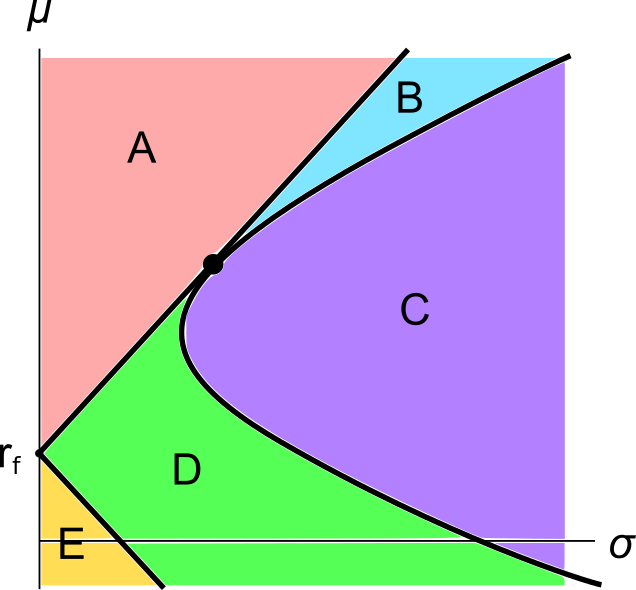# Fight Finance

#### CoursesTagsRandomAllRecentScores

Jan asks you for a loan. He wants $100 now and offers to pay you back$120 in 1 year. You can borrow and lend from the bank at an interest rate of 10% pa, given as an effective annual rate.

Ignore credit risk. Remember:

$$V_0 = \frac{V_t}{(1+r_\text{eff})^t}$$

Will you or Jan's deal?

A firm wishes to raise $20 million now. They will issue 8% pa semi-annual coupon bonds that will mature in 5 years and have a face value of$100 each. Bond yields are 6% pa, given as an APR compounding every 6 months, and the yield curve is flat.

How many bonds should the firm issue?

Harvey Norman the large retailer often runs sales advertising 2 years interest free when you purchase its products. This offer can be seen as a free personal loan from Harvey Norman to its customers.

Assume that banks charge an interest rate on personal loans of 12% pa given as an APR compounding per month. This is the interest rate that Harvey Norman deserves on the 2 year loan it extends to its customers. Therefore Harvey Norman must implicitly include the cost of this loan in the advertised sale price of its goods.

If you were a customer buying from Harvey Norman, and you were paying immediately, not in 2 years, what is the minimum percentage discount to the advertised sale price that you would insist on? (Hint: if it makes it easier, assume that you’re buying a product with an advertised price of \$100).

The coupon rate of a fixed annual-coupon bond is constant (always the same).

What can you say about the income return ($r_\text{income}$) of a fixed annual coupon bond? Remember that:

$$r_\text{total} = r_\text{income} + r_\text{capital}$$

$$r_\text{total, 0 to 1} = \frac{c_1}{p_0} + \frac{p_1-p_0}{p_0}$$

Assume that there is no change in the bond's total annual yield to maturity from when it is issued to when it matures.

Select the most correct statement.

From its date of issue until maturity, the income return of a fixed annual coupon:

The market expects the Reserve Bank of Australia (RBA) to increase the policy rate by 25 basis points at their next meeting.

As expected, the RBA increases the policy rate by 25 basis points.

What do you expect to happen to Australia's exchange rate in the short term? The Australian dollar will:

There are many ways to calculate a firm's free cash flow (FFCF), also called cash flow from assets (CFFA). Some include the annual interest tax shield in the cash flow and some do not.

Which of the below FFCF formulas include the interest tax shield in the cash flow?

$$(1) \quad FFCF=NI + Depr - CapEx -ΔNWC + IntExp$$ $$(2) \quad FFCF=NI + Depr - CapEx -ΔNWC + IntExp.(1-t_c)$$ $$(3) \quad FFCF=EBIT.(1-t_c )+ Depr- CapEx -ΔNWC+IntExp.t_c$$ $$(4) \quad FFCF=EBIT.(1-t_c) + Depr- CapEx -ΔNWC$$ $$(5) \quad FFCF=EBITDA.(1-t_c )+Depr.t_c- CapEx -ΔNWC+IntExp.t_c$$ $$(6) \quad FFCF=EBITDA.(1-t_c )+Depr.t_c- CapEx -ΔNWC$$ $$(7) \quad FFCF=EBIT-Tax + Depr - CapEx -ΔNWC$$ $$(8) \quad FFCF=EBIT-Tax + Depr - CapEx -ΔNWC-IntExp.t_c$$ $$(9) \quad FFCF=EBITDA-Tax - CapEx -ΔNWC$$ $$(10) \quad FFCF=EBITDA-Tax - CapEx -ΔNWC-IntExp.t_c$$

The formulas for net income (NI also called earnings), EBIT and EBITDA are given below. Assume that depreciation and amortisation are both represented by 'Depr' and that 'FC' represents fixed costs such as rent.

$$NI=(Rev - COGS - Depr - FC - IntExp).(1-t_c )$$ $$EBIT=Rev - COGS - FC-Depr$$ $$EBITDA=Rev - COGS - FC$$ $$Tax =(Rev - COGS - Depr - FC - IntExp).t_c= \dfrac{NI.t_c}{1-t_c}$$Assets A, B, M and $r_f$ are shown on the graphs above. Asset M is the market portfolio and $r_f$ is the risk free yield on government bonds. Which of the below statements is NOT correct?

A graph of assets’ expected returns $(\mu)$ versus standard deviations $(\sigma)$ is given in the below diagram.

Each letter corresponds to a separate coloured area. The portfolios at the boundary of the areas, on the black lines, are excluded from each area. Assume that all assets represented in this graph are fairly priced, and that all risky assets can be short-sold.Suppose the yield curve in the USA and Germany is flat and the:

• USD federal funds rate at the Federal Reserve is 1% pa;
• EUR deposit facility at the European Central Bank is -0.4% pa (note the negative sign);
• Spot EUR exchange rate is 1 USD per EUR;
• One year forward EUR exchange rate is 1.011 USD per EUR.

You suspect that there’s an arbitrage opportunity. Which one of the following statements about the potential arbitrage opportunity is NOT correct?

A Malaysian man wishes to convert 1 million Malaysian Ringgit (MYR) into Indian Rupees (IND). The exchange rate is 4.2 MYR per USD and 71 IND per USD. How much is the MYR 1 million worth in IND?